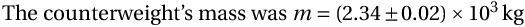# The siunitx Package

Documentation physics Essential for typesetting numbers with units. Use it religiously! \usepackage{siunitx}
The counterweight's mass was $m = \SI{2.34(2)e3}{kg}$


Note: recent versions of siunitx have changed the standard macro to \qty, which interferes with the macro with the same name in the physics package. If you load physics before siunitx, the physics version is retained and you can use \SI{val}{units} as before.

If you need to put something in the numeric part that collides with siunitx's formatter, you can still use \unit{units} to set the units part properly.

• For angles, use \ang{1;2;3} to get 1°2'3''
• \num[options]{number} for numerical values: \num[color=blue]{1.234e7}
• \unit[<options>]{<unit>} for just the unit portion. Separate units with periods: \unit{kg.m/s^2}
• \complexnum[]{], as in \complexnum{1+-2i}